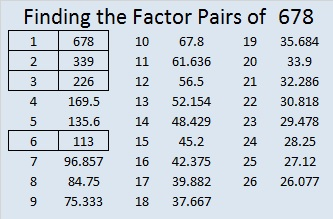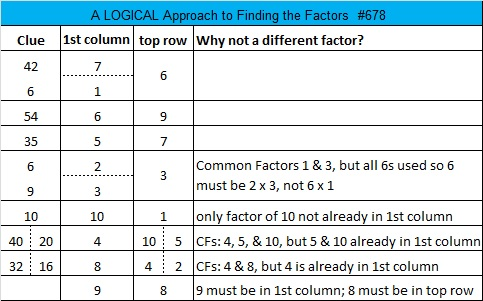# 678 and Level 5

678 is made from 3 consecutive numbers so it is divisible by 3. The middle number of those 3 consecutive numbers is not divisible by 3, so 678 is NOT divisible by 9.

678 is the hypotenuse of  Pythagorean triple 90-672-678. What is the greatest common factor of those 3 numbers?Print the puzzles or type the solution on this excel file: 10 Factors 2015-11-09

—————————————————————————————————

• 678 is a composite number.
• Prime factorization: 678 = 2 x 3 x 113
• The exponents in the prime factorization are 1, 1, and 1. Adding one to each and multiplying we get (1 + 1)(1 + 1)(1 + 1) = 2 x 2 x 2 = 8. Therefore 678 has exactly 8 factors.
• Factors of 678: 1, 2, 3, 6, 113, 226, 339, 678
• Factor pairs: 678 = 1 x 678, 2 x 339, 3 x 226, or 6 x 113
• 678 has no square factors that allow its square root to be simplified. √678 ≈ 26.038433.—————————————————————————————————